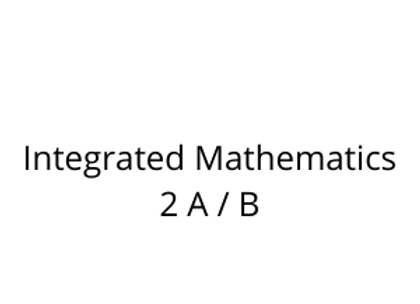# Integrated Mathematics 2 A / B

\$395.00Price
`Integrated Math is a two-semester, comprehensive collection of mathematical concepts designed to give you a deeper understanding of the world around you. It includes ideas from algebra, geometry, probability and statistics, and trigonometry, and teaches these subjects as interrelated disciplines. It’s likely that you’ve been studying some form of integrated math since elementary school. In Integrated Math 2A, you will begin with polynomial expressions, including rational expressions. You will learn about quadratic equations and inequalities and solve them to find answers to real-world math problems. Finally, you will use this knowledge to examine polynomial functions.  In Integrated Math 2B, you will study the connections between algebra and geometry. You will learn about functions and use them to solve real-world math problems. You will study data collection methods, and you will use different types of data plots to represent and analyze statistical data. You will learn about geometric theorems and rules and write proofs to support them. You will also explore congruency and similarity of triangles.`